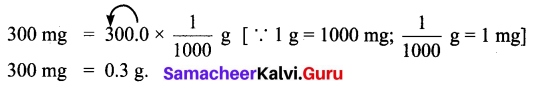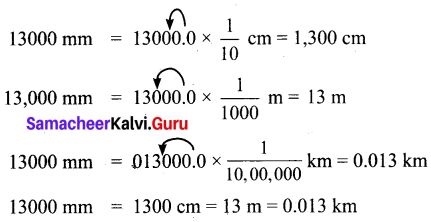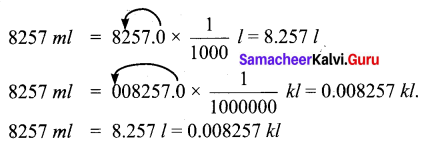## Tamilnadu Samacheer Kalvi 6th Maths Solutions Term 2 Chapter 2 Measurements Ex 2.1

Question 1.
Fill in the blanks.
(i) 250 ml + $$\frac{1}{2}$$ ml = _____ l.
(ii) 150 kg 200 g + 55 kg 750 g = ____ kg ____ g.
(iii) 20 l – 1 l 500 ml = ____ l ___ ml
(iv) 450 ml × 5 = ____ l ____ ml.
(v) 50 Kg ÷ 100 g = ______
Solution:
(i) $$\frac{3}{4}$$ l
(ii) 205 kg 950 g
(iii) 18 l 500 ml
(iv) 2l 250 ml
(v) 500

Question 2.
True or False
(i) Pugazhenthi ate 100 g of nuts which is equal to 0.1 kg.
(ii) Meena bow it 250 ml of butter milk which is equal to 2.5 l.
(iii) Karkuzhali’s bag 1 kg 250 g and poong- kodi’s bag 2 kg 750 g. The total weight of their bags 4 kg.
(iv) Vanmathi bought 4 books each weighing 500 g. Total weight of 4 books is 2 kg.
(v) Gayathiri bought 1 kg of birthday cake. She shared 450 g with her friends.
The weight of cake remaining is 650 g
Solution:
(i) True
(ii) False
(iii) True
(iv) True
(v) False

Question 3.
Convert into indicated units:
(i) 10 l and 5 ml into ml
(ii) 4 km and 300 m into m
(iii) 300 mg into g
Solution:
(i) 10 l and 5 ml
= 10 × 1000 ml + 5 ml
= 10,000 ml + 5 ml [∴ 1l = 1000 ml]
= 10,005 ml.
∴ 10 l and 5 ml = 10,005 ml.
(ii) 4 km and 300 m into m.
4 km and 300 m
= 4 × 1000 m + 300 m
= 4000 m + 300 m [∴ 1 km = 1000 m]
= 4300 m
∴ 4 km and 300 m = 4300 m
(iii) 300 mg into g.Question 4.
Convert into higher units:
(i) 13000 mm (km, m, cm)
(ii) 8257 ml (kl, l)
Solution:
(i) 13000 mm (km, m, cm)
(ii) 8257 ml (kl, l)Question 5.
Convert into lower units:
(i) 15 km (m, cm, mm)
Solution:
15 km = 15 × 1000 m = 15000 m
15 km = 15 × 100000 cm
= 1500000 cm
15 km = 15 × 1000000 mm
= 15000000 mm

(ii) 12 kg (g, mg)
Solution:
12 kg = 12 × 1000 g
= 12000 g
12 kg = 12 × 1000000 mg
= 12000000 mg

Question 6.
Compare and put > or < or = in the following:
(i) 800 g + 150 g ____ 1 kg
(ii) 600 ml + 400 ml ____ 1 l
(iii) 6 m 25 cm ____ 600 cm + 25 cm
(iv) 88 cm ____ 8 m 8 cm
(v) 55 g ____ 550 mg
Solution:
(i) 800 g + 150g < 3kg
(ii) 600 ml + 400 ml = 1 l
(iii) 6 m 25 cm = 600 cm + 25 cm
(iv) 88 cm < 8 m 8 cm
(v) 55 g > 550 mg

Question 7.
Geetha brought 2 l and 250 ml of water in a bottle. Her friend drank 300 ml from it. How much of water is remaining in the bottle?
Solution:
Total Capacity of water = 2 l 250 ml
= (2 x 1000 + 250) l
= 2000 + 250 ml
= 2250 ml
Water Consumed = 300 ml
Water remaining in the bottle = (2250 – 300) ml
= 1950 ml
= 1 litre 950 mlQuestion 8.
Thenmozhi’s height is 1.25 m now. She grows for 5 cm every year. What would be her height after 6 years?
Solution:
Thenmozhi’s present height = 1.25 m
Rate of growth per year = 5 cm
Her growth in 6 years = 5 cm × 6 = 30 cm.
After 6 years her height = 1.25 m + 30 cm
= 1.25 × 100 + 30 cm
= 125 + 30 cm
= 155 cm.
∴ After 6 years Thenmozhi’s height will be 155 cm.

Question 9.
Priya bought 22 $$\frac{1}{2}$$ kg of onion/ Krishna bought 18 $$\frac{3}{4}$$ kg of onion and sethu bought 9 kg 250 g of onion, what is the total weight of onion did they buy?
Solution:
Total weight of onion bought
= 22 $$\frac{1}{2}$$ + 18 $$\frac{3}{4}$$ + 9 $$\frac{1}{4}$$ kg
= 22 kg 500 g + 18 kg 750 g + 9 kg 250 g
= 49 kg 1500 g
= 50 kg 500 g

Question 10.
Maran walks 1.5 km every day to reach the school while Mahizhan walks 1400 m. Who walks more distance and by how much?
Solution:
Distance which Maran walks = 1.5 km = 1.5 × 1000 m = 1500 m
The distance which Mahizhan walks = 1400 m.
Here 1500 > 1400
∴ Difference = 1500 – 1400 = 100 m.
∴ Maran walks more distance = 100 m.

Question 11.
In a JRC one day camp, 150 gm of rice and 15 ml oil are needed for a student. If there are 40 students to attend the camp how much of rice and oil are needed?
Solution:
Rice needed for a student = 150 gm
Rice needed for 40 students = 40 × 150 gm = 6000 gm = 6 kg
Oil needed for a student = 15 ml
Oil needed for 40 students = 40 × 15 ml = 600 ml

Question 12.
In a school, 200 litres of lemon juice is prepared. If 250 ml lemon juice is given to each student, how many students get the juice?
Solution:
Total lemon juice prepared = 200 l = 200 × 1000 ml = 2,00,000 ml.
∴ Quantity of Lemon juice given to one student = 250 ml.
∴ Number of students can get = $$\frac{2,00,000}{250}$$ = 800
∴ 800 students can get the lemon juice.Question 13.
How many glasses of the given capacity will fill a 2 litre jug?
(i) 100 ml ……….
(ii) 50 ml ……….
(iii) 500 ml ………
(iv) 1 l ………
(v) 250 ml ………
Solution:
(i) 20
(ii) 40
(iii) 4
(iv) 2
(v) 8

Objective Type Questions

Question 14.
9 m 4 cm is equal to _____
(a) 94 cm
(b) 904 cm
(c) 9.4 cm
(d) 0.94 cm
Solution:
(b) 904 cm

Question 15.
1006 g is equal to ………
(i) 1 kg 6 g
(ii) 10 kg 6 g
(iii) 100 kg 6 g
(iv) 1 kg 600 g
Solution:
(i) 1 kg 6 g

Question 16.
Every day 150 l of water is sprayed in the garden. Water sprayed in a week is ____
(a) 700 l
(b) 1000 l
(c) 950 l
(d) 1050 l
Solution:
(d) 1050 l

Question 17.
Which is the greatest? 0.007 g, 70 mg, 0.07 cg ……….
(i) 0.07 cg
(ii) 0.007 g
(iii) 70 mg
(iv) all are equal
Solution:
(iii) 70 mgQuestion 18.
7 km – 4200 m is equal to
(a) 3 km 800 m
(b) 2 km 800 m
(c) 3 km 200 rn
(d) 2 km 200 m
Solution:
(b) 2 km 800 m